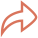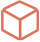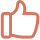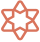👆点击关注｜设为星标｜干货速递👆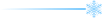1. 从一个例子（贷款前，评估个人能否偿还）出发，怎样决策。

2. 分析算法原理、思路形成的过程。

3. 扩展决策树衍生的高级版本进行简要的介绍。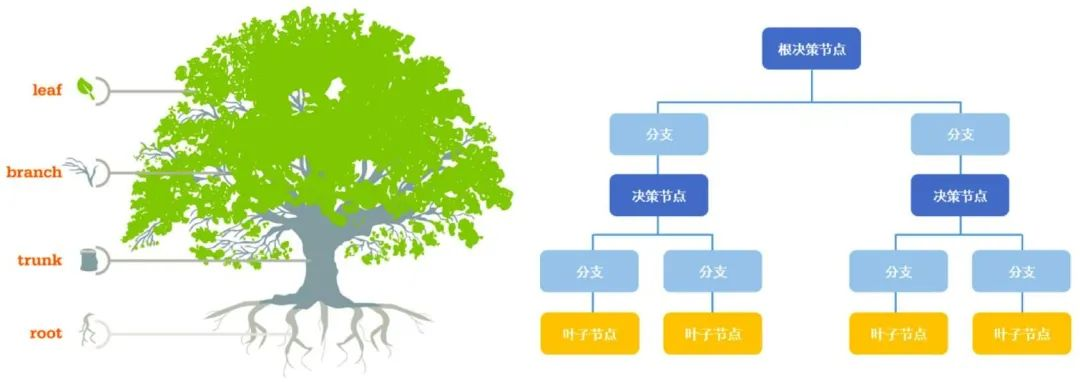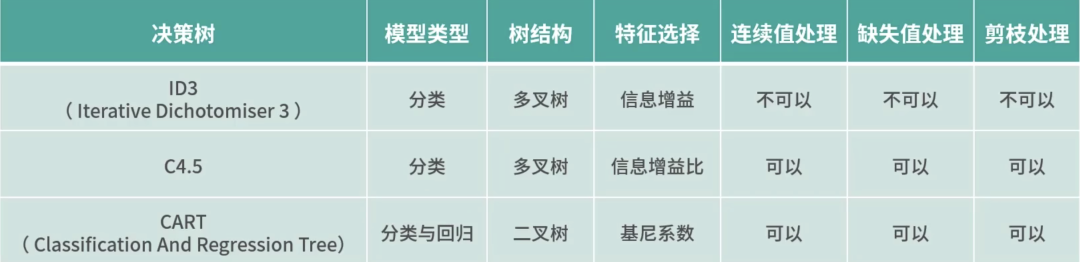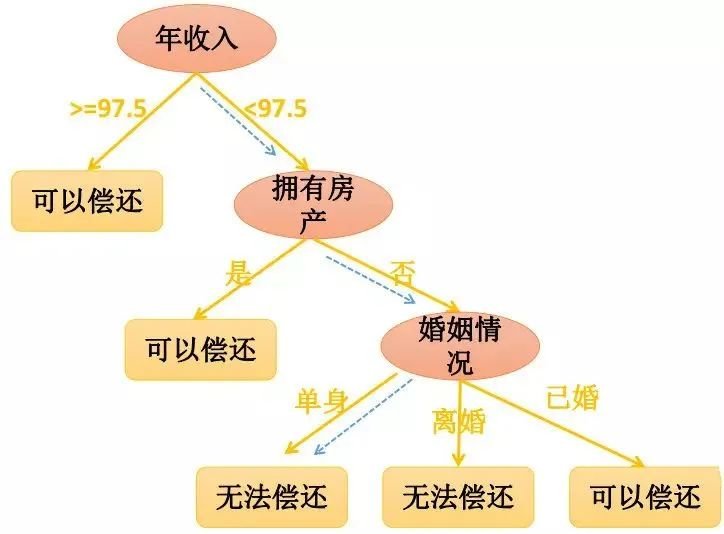（图片来源于网络）1. 非常直观，可解释性极强。

2. 预测速度比较快（由条件判断即可）。

3. 既可以处理离散值也可以出来连续值，还可以处理缺失值。

1. 容易过拟合。

2. 需要处理样本不均衡的问题。

3. 样本的变化会引发树结构巨变。

1. 预剪枝：在决策树构建之初就设定一个阈值，当分裂节点的熵阈值小于设定值的时候就不再进行分裂了。

2. 后剪枝：在决策树已经构建完成后，再根据设定的条件来判断是否要合并一些中间节点，使用叶子节点来代替。``````# sklearn 数据集
from sklearn import datasets
# 引入决策树算法包
from sklearn.tree import DecisionTreeClassifier
# 矩阵运算库numpy
import numpy as np

# 设置随机种子，可以保证每次产生的随机数是一样的
np.random.seed(0)
# 获取鸢尾花数据集
iris_x = iris.data # 数据部分
iris_y = iris.target # 类别部分``````

``````# 从150条数据中选择140条作为训练集，10条作为测试集。
# permutation接收一个数作为参数（这里为数据集长度150）。产生一个0-149乱序一维数组
randomarr = np.random.permutation(len(iris_x))
iris_x_train = iris_x[randomarr[:-10]] # 训练集数据
iris_y_train = iris_y[randomarr[:-10]] # 训练集标签
iris_x_test = iris_x[randomarr[-10:]] # 测试集数据
iris_y_test = iris_y[randomarr[-10:]] # 训练集标签

clf = DecisionTreeClassifier(max_depth=4)
# 调用该对象的训练方法，主要接收两个参数：训练数据集及其类别标签
clf.fit(iris_x_train,iris_y_train)``````

``````# 引入画图相关的包
from IPython.display import  Image
from sklearn import tree
# dot是一个程序化生成流程图的简单语言
import pydotplus
dot_data = tree.export_graphviz(
clf,
out_file=None,
feature_names = iris.feature_names,
class_names = iris.target_names,
filled = True,
rounded = True,
special_characters=True)

graph = pydotplus.graph_from_dot_data(dot_data)
Image(graph.create_png())``````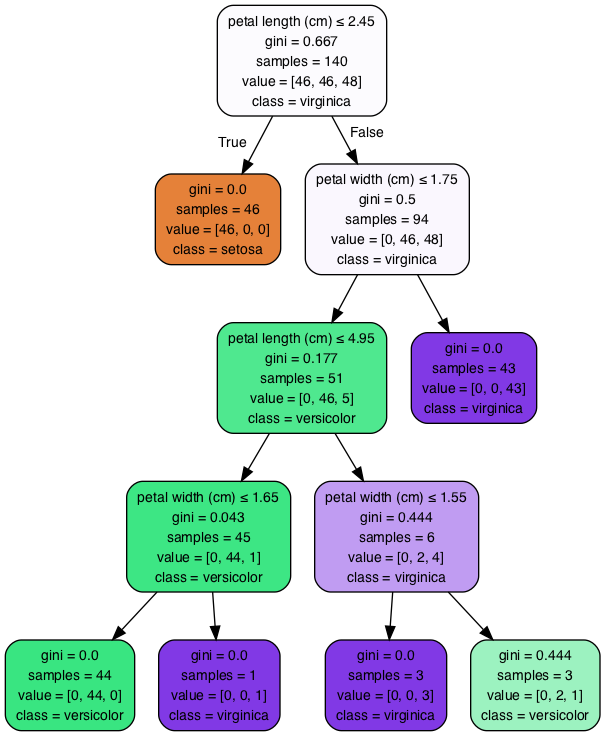``````# 调用预测方法，主要接收一个参数：测试数据集
iris_y_predict = clf.predict(iris_x_test)
# 计算各预测样本预测的概率值
probility = clf.predict_proba(iris_x_test)
# 计算出准确率
score = clf.score(iris_x_test,iris_y_test,sample_weight=None)

# 输出预测结果
print("iris_y_predict=",iris_y_predict)
# 输出原始结果
print("iris_y_test=",iris_y_test)
# 输出准确率
print("Accuracy:",score)``````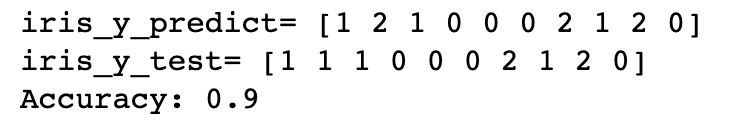GBDT：GBDT构建的构建的多棵树之间是有联系的，每个分类器在上一轮分类器的残差基础上进行训练。

XGBoost：优化了GBDl里面的求解过程，并加入了很多工程上的优化项目。END